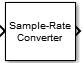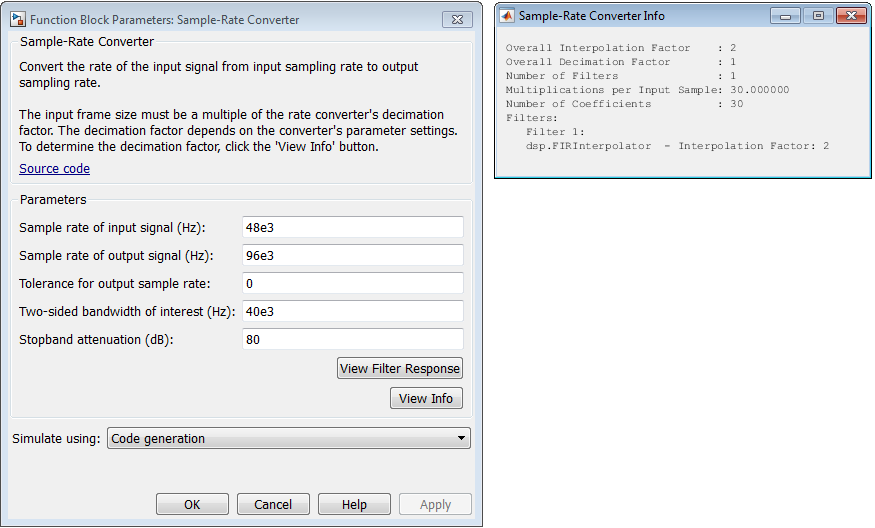# Sample-Rate Converter

Multistage sample-rate conversion

•Libraries:
DSP System Toolbox / Signal Operations

## Description

The Sample-Rate Converter block implements a multistage FIR sample-rate converter. The multistage FIR converter converts the rate of each channel of the input signal from the input sample rate to the output sample rate. Multistage implementations minimize the amount of computation required for the sample-rate conversions by first reducing the sample rate of the input signal. Next, the block determines the optimal number of decimators and interpolators based on the parameters you specify in the block dialog box. The block then accordingly designs filters in the individual stages.

This block supports SIMD code generation. For details, see Code Generation.

## Ports

### Input

expand all

Specify the input signal as a real or a complex-valued scalar, vector, or a matrix.

The block treats each column of a two-dimensional input signal as a separate channel. If the input is a two-dimensional signal, the first dimension represents the channel length (or frame size), and the second dimension represents the number of channels. If the input is a one-dimensional signal, then the block interprets the signal as having a single channel.

When you input a variable-size signal (frame length changes during simulation), the frame length of the signal can be arbitrary, that is, the input frame length does not have to be a multiple of the decimation factor. When you input a fixed-size signal (frame length does not change during simulation), the frame length can be arbitrary only when you select the Allow arbitrary frame length for fixed-size input signals parameter.

To determine the decimation factor, click the button in the block dialog box.

Data Types: `single` | `double`
Complex Number Support: Yes

### Output

expand all

The Sample-Rate Converter block outputs a signal that has the same data type and complexity as the input signal.

The output signal dimensions depend on the input signal dimensions, the overall interpolation and decimation factors of the Sample-Rate Converter block, and whether you select the Allow arbitrary frame length for fixed-size input signals parameter. You can determine the overall interpolation factor L and the decimator factor M by clicking the button in the block dialog box.

This table provides more details on the dimensions of the output signal when you input a fixed-size input signal.

Fixed-Size Input Signal

Input SignalOutput Signal Dimensions

P-by-1 column vector, where P is a multiple of the decimation factor M

(LP/M)-by-1, where L is the interpolation factor

P-by-1 column vector, where P is not a multiple of the decimation factor M

`ceil`(LP/M)-by-1 when you select Allow arbitrary frame length for fixed-size input signals.

If you do not select Allow arbitrary frame length for fixed-size input signals, the block errors.

P-by-Q matrix, where P is a multiple of the decimation factor M

(LP/M)-by-Q

P-by-Q matrix, where P is not a multiple of the decimation factor M

`ceil`(LP/M)-by-Q when you select Allow arbitrary frame length for fixed-size input signals.

If you do not select Allow arbitrary frame length for fixed-size input signals, the block errors.

This table gives more details on the dimensions of the output signal when you input a variable-size input signal. When you input a variable-size signal (frame length changes during simulation), the Allow arbitrary frame length for fixed-size input signals parameter is visible in the block dialog box but does not have any impact on the input frame length. You can input a variable-size signal of any frame length even if you do not select the Allow arbitrary frame length for fixed-size input signals parameter.

Variable-Size Input Signal

Input SignalOutput Signal Dimensions

P-by-Q

`ceil`(LP/M)-by-Q

Data Types: `single` | `double`
Complex Number Support: Yes

## Parameters

expand all

Specify the sample rate of the input signal as a positive scalar in Hz. The input sample rate must be greater than the bandwidth of interest.

Specify the sample rate of the output signal as a positive scalar in Hz. The output sample rate must be greater than the bandwidth of interest.

Specify the maximum tolerance for the output sample rate as a positive scalar in the range [`0`,`0.5`]. The default is `0`.

The actual output sample rate varies but is within the specified range. For example, if you set the Tolerance for output sample rate to 0.01, then the actual output sample rate is in the range given by sample rate of output signal ± 1%. This flexibility allows for a simpler filter design.

Specify the two-sided bandwidth of interest (after the rate of conversion) as a positive scalar in Hz.

Specify the minimum amount of attenuation for aliased components in the stopband as a positive scalar in dB. This parameter is the minimum amount by which the block attenuates any aliasing involved in the process.

Since R2023a

Specify whether fixed-size input signals (whose size does not change during simulation) can have an arbitrary frame length, where the frame length does not have to be a multiple of the decimation factor. The block uses this parameter only for fixed-size input signals and ignores it if the input data varies in size during simulation.

When the input signal is a variable-size signal, the signal can have arbitrary frame length, that is, the frame length does not have to be a multiple of the decimation factor.

For fixed-size input signals, if you:

• Select the Allow arbitrary frame length for fixed-size input signals parameter, the frame length of the signal does not have to be a multiple of the decimation factor. If the input is not a multiple of the decimation factor, then the output is generally a variable-size signal. Therefore, to support arbitrary input size, the block must also support variable-size operations, which you can enable by selecting the Allow arbitrary frame length for fixed-size input signals parameter.

• Clear the Allow arbitrary frame length for fixed-size input signals parameter, the input frame length must be a multiple of the decimation factor.

Opens the Filter Visualization Tool FVTool and displays the magnitude/phase response of the Sample-Rate Converter. The response is based on the block parameters. FVTool updates when you change the parameters.To update the magnitude response while FVTool is running, modify the parameters in the dialog box and click .

Click on the button to display the filter information of the Sample-Rate Converter block:

• `Overall Interpolation Factor`

• `Overall Decimation Factor`

• `Number of Filters`

• `Multiplication per Input Sample`

• `Number of Coefficients`

• `Filters`The button brings the functionality of the `info` function into the Simulink® environment.

Type of simulation to run. You can set this parameter to:

• `Code generation` (default)

Simulate model using generated C code. The first time you run a simulation, Simulink generates C code for the block. The C code is reused for subsequent simulations, as long as the model does not change. This option requires additional startup time but provides faster simulation speed than ```Interpreted execution```.

• `Interpreted execution`

Simulate model using the MATLAB®  interpreter. This option shortens startup time but has slower simulation speed than ```Code generation```.

## Block Characteristics

 Data Types `double` | `single` Direct Feedthrough `no` Multidimensional Signals `no` Variable-Size Signals `yes` Zero-Crossing Detection `no`

## Algorithms

• The general multistage sample rate converter performs a multistage decimation, a single-stage sample rate conversion, and a multistage interpolation. Actual designs include at most two of these steps.

• The procedure automatically determines the optimal number of decimation or interpolation stages. In special cases, the algorithm can perform a decimation or interpolation in a single stage.

• The algorithm always attempts to start by reducing the sample rate. This decreases the amount of computation required. The decimation step ensures that the intermediate sample rate does not go below the bandwidth of interest and that the block does not filter out any information.

• Each individual stage uses halfband or Nyquist filters to minimize the number of nonzero coefficients.

• The algorithm allows transition-band aliasing because it decreases the implementation cost. It keeps the signal within the bandwidth of interest alias free up to the value specified by the `StopbandAttenuation` property.

## Version History

Introduced in R2015b

expand all